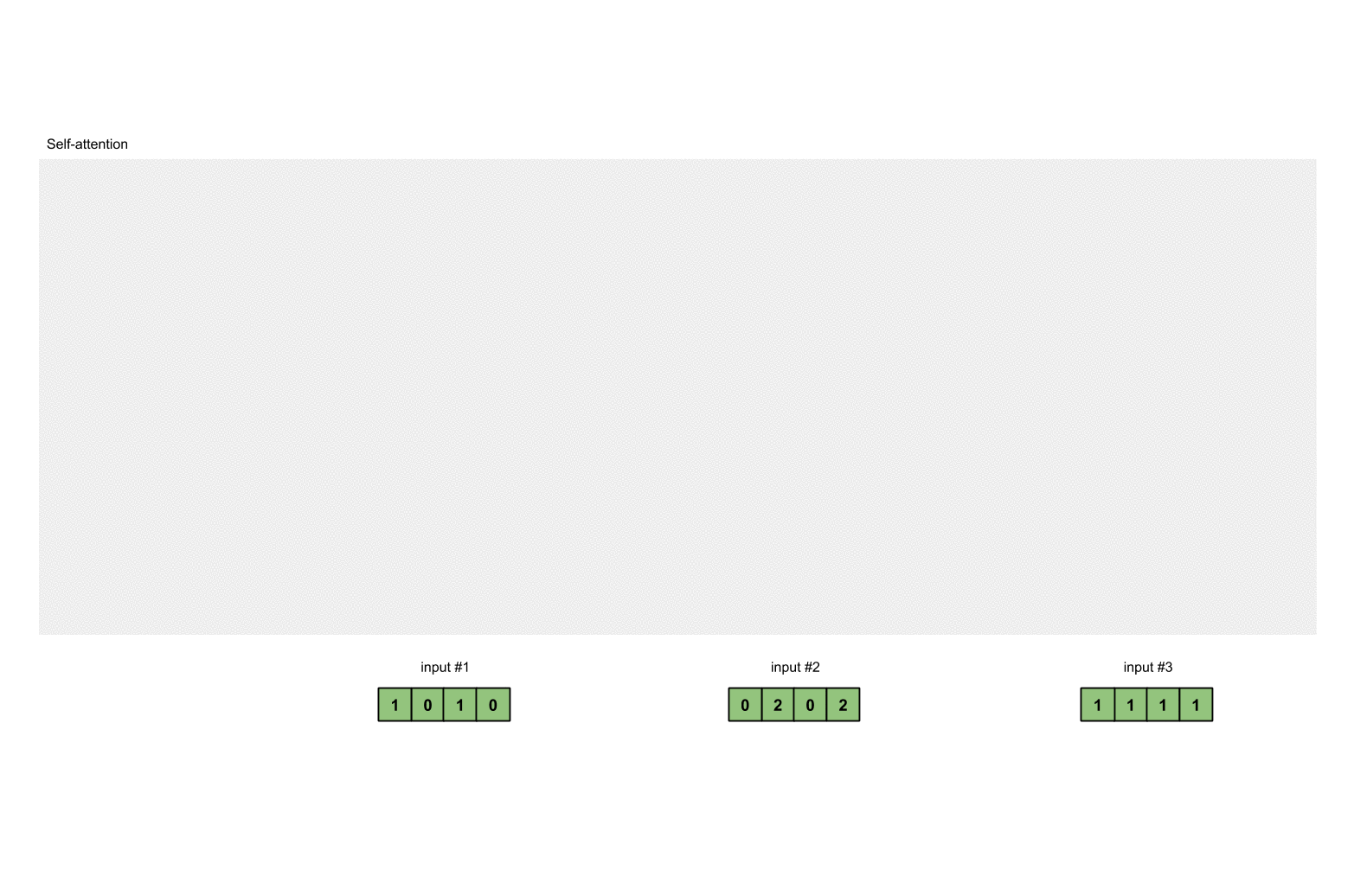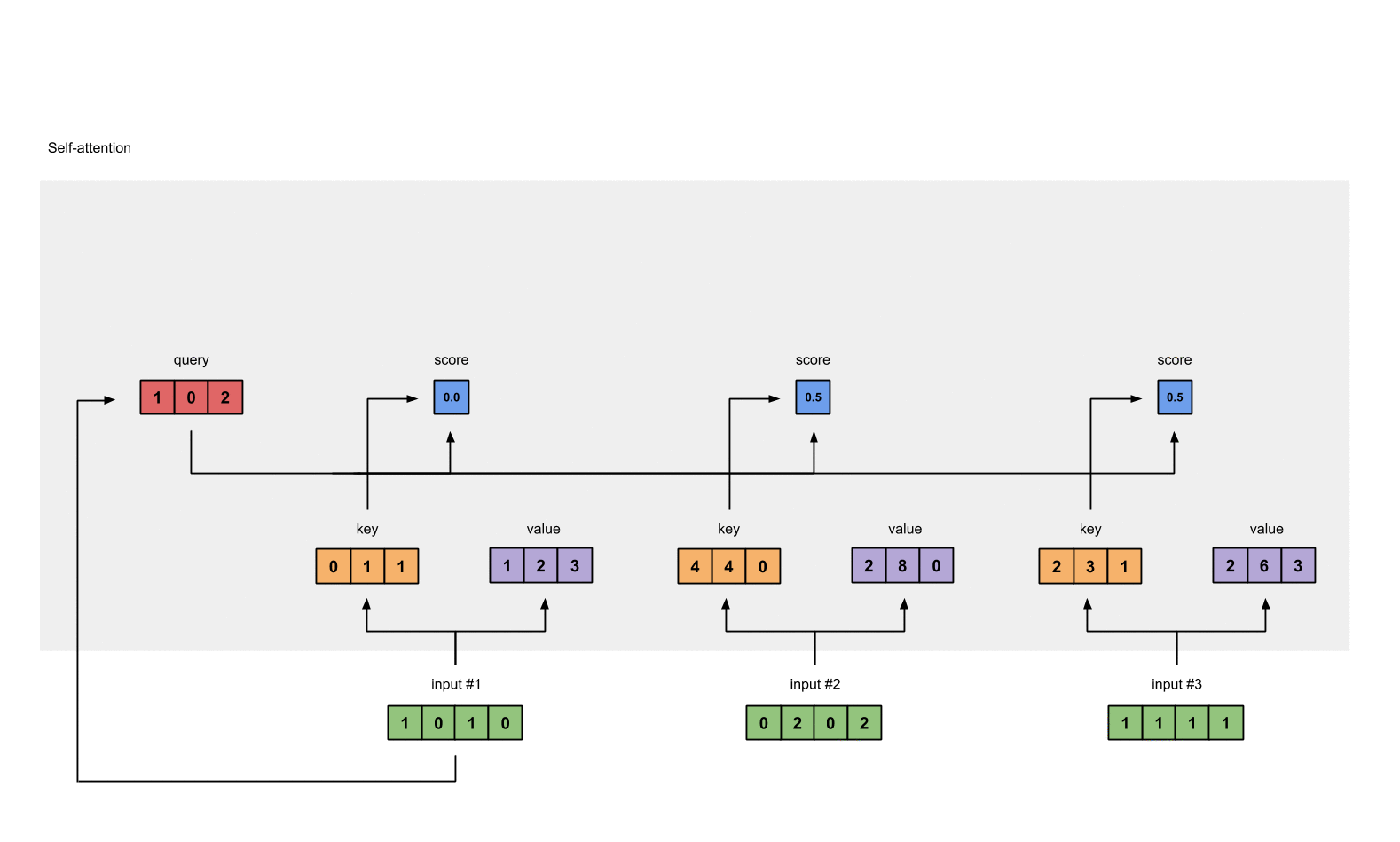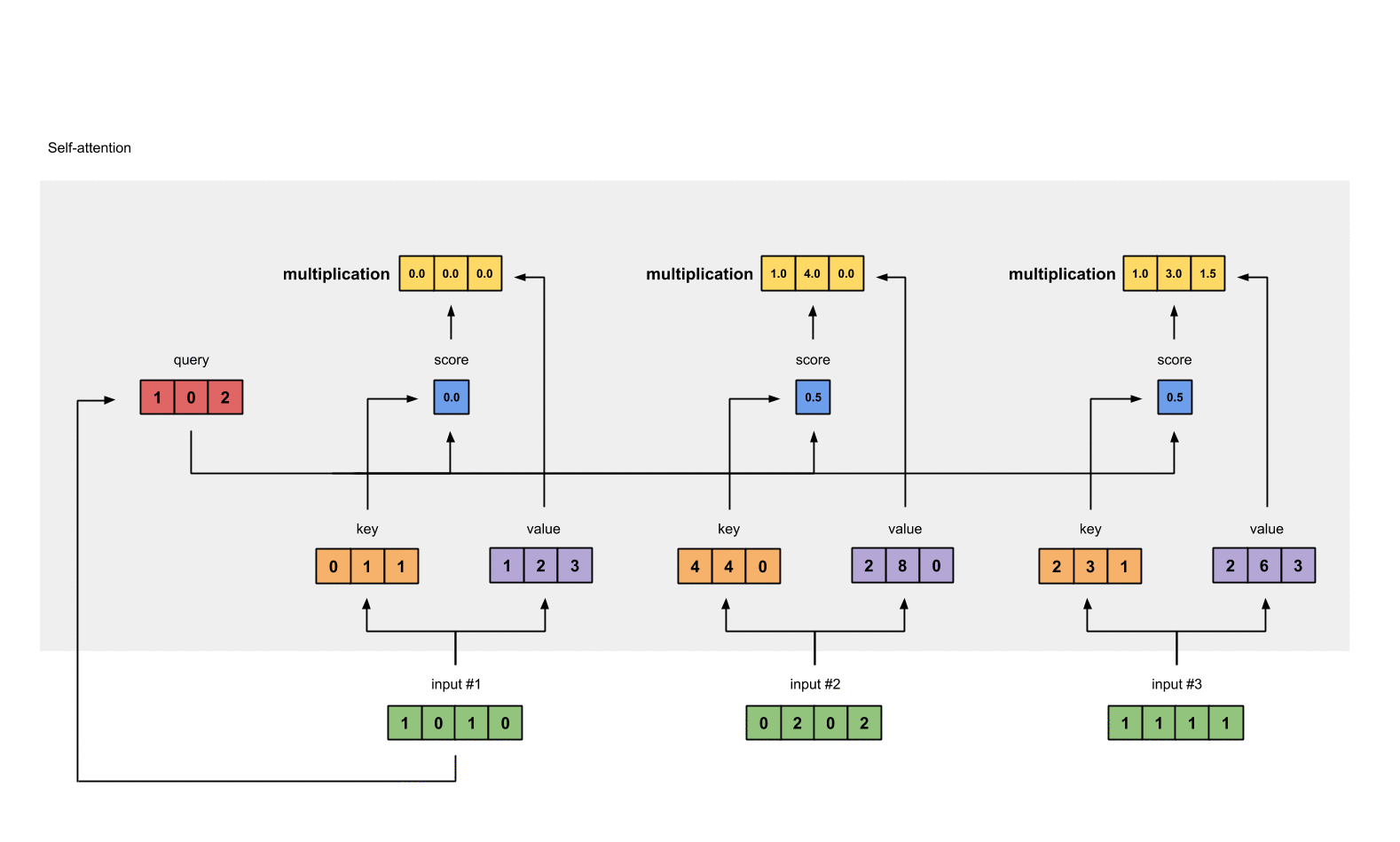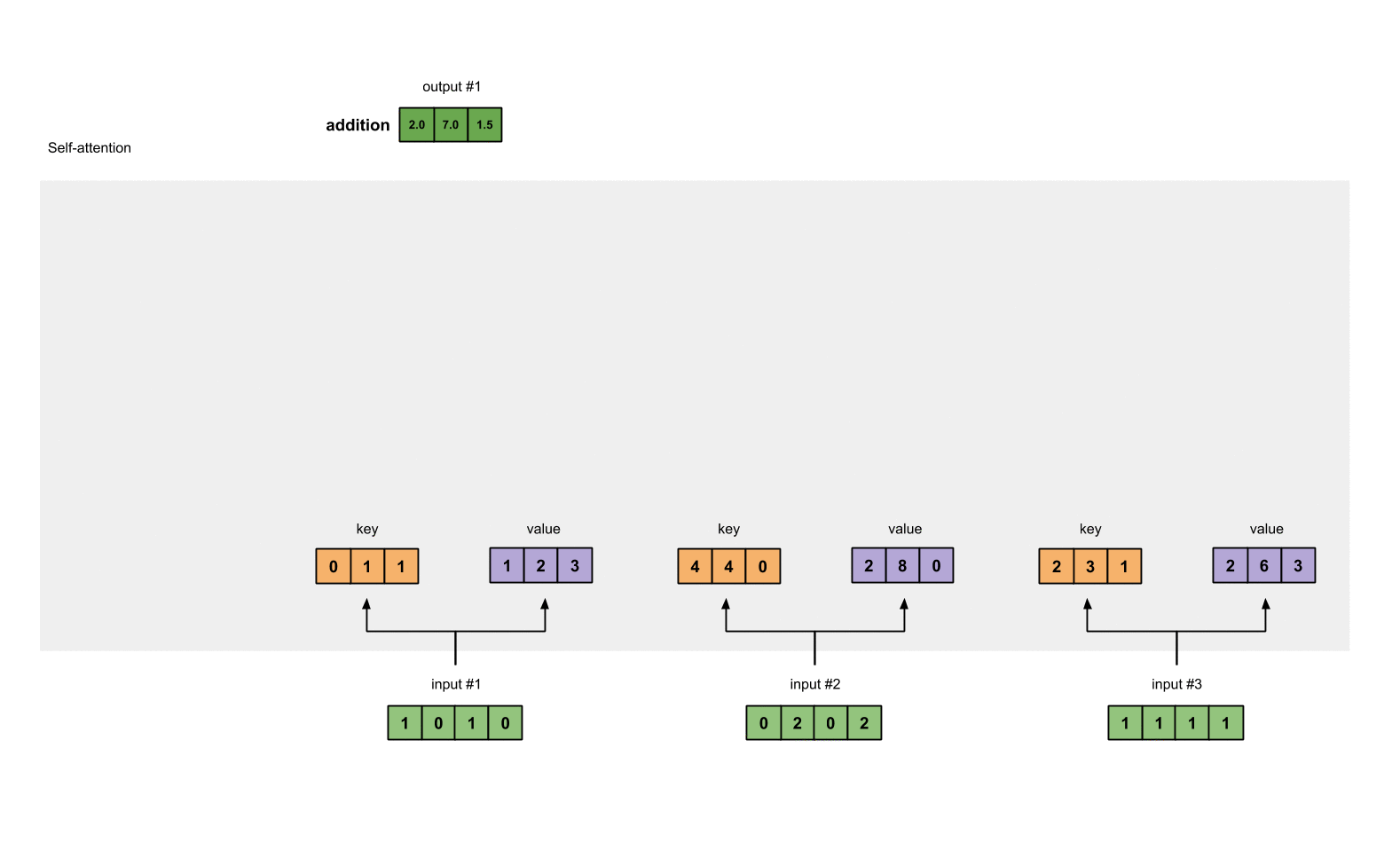###### Evaluation metrics for Multi-Label Classification with Python codes
2022-06-19###### A tutorial on Pandas apply, applymap, map, and transform
2022-06-23

What do BERT, RoBERTa, ALBERT, SpanBERT, DistilBERT, SesameBERT, SemBERT, SciBERT, BioBERT, MobileBERT, TinyBERT and CamemBERT all have in common? And I’m not looking for the answer “BERT”.

Answer: self-attention. We are not only talking about architectures bearing the name “BERT’ but, more correctly, Transformer-based architectures. Transformer-based architectures, which are primarily used in modeling language understanding tasks, eschew recurrence in neural networks and instead trust entirely on self-attention mechanisms to draw global dependencies between inputs and outputs. But what’s the math behind this?

That’s what we’re going to find out today. The main content of this post is to walk you through the mathematical operations involved in a self-attention module. By the end of this article, you should be able to write or code a self-attention module from scratch.

This article does not aim to provide the intuitions and explanations behind the different numerical representations and mathematical operations in the self-attention module. It also does not seek to demonstrate the why’s and how exactly of self-attention in Transformers. Note that the difference between attention and self-attention is also not detailed in this article.

A Colab version can be found here

## What is self-attention?

If you think that self-attention is similar, the answer is yes! They fundamentally share the same concept and many common mathematical operations. A self-attention module takes in n inputs and returns outputs. What happens in this module? In layman’s terms, the self-attention mechanism allows the inputs to interact with each other (“self”) and find out to who they should pay more attention (“attention”). The outputs are aggregates of these interactions and attention scores.

## Illustrations

The illustrations are divided into the following steps:

1. Prepare inputs
2. Initialize weights
3. Derive keyquery, and value
4. Calculate attention scores for Input 1
5. Calculate softmax
6. Multiply scores with values
7. Sum weighted values to get Output 1
8. Repeat steps 4–7 for Input 2 & Input 3

Note
In practice, the mathematical operations are vectorized, i.e. all the inputs undergo the mathematical operations together. We’ll see this later in the Code section.

Step 1: Prepare inputs

We start with 3 inputs for this tutorial, each with dimension 4.

```Input 1: [1, 0, 1, 0]
Input 2: [0, 2, 0, 2]
Input 3: [1, 1, 1, 1]
```

Step 2: Initialise weights

Every input must have three representations (see diagram below). These representations are called key (orange), query (red), and value (purple). For this example, let’s take that we want these representations to have a dimension of 3. Because every input has a dimension of 4, each set of the weights must have a shape of 4×3.

Note
We’ll see later that the dimension of
value is also the output dimension.Fig. 1.2: Deriving key, query, and value representations from each input

To obtain these representations, every input (green) is multiplied with a set of weights for keys, a set of weights for queries (I know that’s not the correct spelling), and a set of weights for values. In our example, we initialize the three sets of weights as follows.

Weights for key:

```[[0, 0, 1],
[1, 1, 0],
[0, 1, 0],
[1, 1, 0]]
```

Weights for query:

```[[1, 0, 1],
[1, 0, 0],
[0, 0, 1],
[0, 1, 1]]
```

Weights for value:

```[[0, 2, 0],
[0, 3, 0],
[1, 0, 3],
[1, 1, 0]]
```

Notes
In a neural network setting, these weights are usually small numbers, initialized randomly using an appropriate random distribution like Gaussian, Xavier, and Kaiming distributions. This initialization is done once before training.

Step 3: Derive key, query, and value

Now that we have the three sets of weights, let’s obtain the keyquery, and value representations for every input.

Key representation for Input 1:

```               [0, 0, 1]
[1, 0, 1, 0] x [1, 1, 0] = [0, 1, 1]
[0, 1, 0]
[1, 1, 0]
```

Use the same set of weights to get the key representation for Input 2:

```               [0, 0, 1]
[0, 2, 0, 2] x [1, 1, 0] = [4, 4, 0]
[0, 1, 0]
[1, 1, 0]
```

Use the same set of weights to get the key representation for Input 3:

```               [0, 0, 1]
[1, 1, 1, 1] x [1, 1, 0] = [2, 3, 1]
[0, 1, 0]
[1, 1, 0]
```

A faster way is to vectorize the above operations:

```               [0, 0, 1]
[1, 0, 1, 0]   [1, 1, 0]   [0, 1, 1]
[0, 2, 0, 2] x [0, 1, 0] = [4, 4, 0]
[1, 1, 1, 1]   [1, 1, 0]   [2, 3, 1]
```

Let’s do the same to obtain the value representations for every input:

```               [0, 2, 0]
[1, 0, 1, 0]   [0, 3, 0]   [1, 2, 3]
[0, 2, 0, 2] x [1, 0, 3] = [2, 8, 0]
[1, 1, 1, 1]   [1, 1, 0]   [2, 6, 3]
```

and finally the query representations:

```               [1, 0, 1]
[1, 0, 1, 0]   [1, 0, 0]   [1, 0, 2]
[0, 2, 0, 2] x [0, 0, 1] = [2, 2, 2]
[1, 1, 1, 1]   [0, 1, 1]   [2, 1, 3]
```

Notes
In practice, a
bias vector may be added to the product of matrix multiplication.

Step 4: Calculate attention scores for Input 1

To obtain attention scores, we start with taking a dot product between Input 1’s query (red) with all keys (orange), including itself. Since there are 3 key representations (because we have 3 inputs), we obtain 3 attention scores (blue).

```            [0, 4, 2]
[1, 0, 2] x [1, 4, 3] = [2, 4, 4]
[1, 0, 1]
```

Notice that we only use the query from Input 1. Later we’ll work on repeating this same step for the other queries.

Note
The above operation is known as
dot product attention, one of the several score functionsOther score functions include scaled dot product and additive/concat.

Step 5: Calculate softmax

Take the softmax across these attention scores (blue).

```softmax([2, 4, 4]) = [0.0, 0.5, 0.5]
```

Note that we round off to 1 decimal place here for readability.

Step 6: Multiply scores with valuesFig. 1.6: Derive weighted value representation (yellow) from multiply value (purple) and score (blue)

The softmaxed attention score for each input (blue) is multiplied by its corresponding value (purple). This results in 3 alignment vectors (yellow). In this tutorial, we’ll refer to them as weighted values.

```1: 0.0 * [1, 2, 3] = [0.0, 0.0, 0.0]
2: 0.5 * [2, 8, 0] = [1.0, 4.0, 0.0]
3: 0.5 * [2, 6, 3] = [1.0, 3.0, 1.5]
```

Step 7: Sum weighted values to get Output 1Fig. 1.7: Sum all weighted values (yellow) to get Output 1 (dark green)

Take all the weighted values (yellow) and sum them element-wise:

```  [0.0, 0.0, 0.0]
+ [1.0, 4.0, 0.0]
+ [1.0, 3.0, 1.5]
-----------------
= [2.0, 7.0, 1.5]
```

The resulting vector [2.0, 7.0, 1.5] (dark green) is Output 1, which is based on the query representation from Input 1 interacting with all other keys, including itself.

Step 8: Repeat for Input 2 & Input 3

Now that we’re done with Output 1, we repeat Steps 4 to 7 for Output 2 and Output 3.Fig. 1.8: Repeat previous steps for Input 2 & Input 3

Notes
The dimension of query and key must always be the same because of the dot product score function. However, the dimension of value may be different from query and key. The resulting output will consequently follow the dimension of value.

## 2. Code

Here is the code in PyTorch, a popular deep learning framework in Python. To enjoy the APIs for `@` operator, `.T` and `None` indexing in the following code snippets, make sure you’re on Python≥3.6 and PyTorch 1.3.1.

Step 1: Prepare inputs

```import torch

x = [
[1, 0, 1, 0], # Input 1
[0, 2, 0, 2], # Input 2
[1, 1, 1, 1]  # Input 3
]
x = torch.tensor(x, dtype=torch.float32)
```

Step 2: Initialise weights

```w_key = [
[0, 0, 1],
[1, 1, 0],
[0, 1, 0],
[1, 1, 0]
]
w_query = [
[1, 0, 1],
[1, 0, 0],
[0, 0, 1],
[0, 1, 1]
]
w_value = [
[0, 2, 0],
[0, 3, 0],
[1, 0, 3],
[1, 1, 0]
]
w_key = torch.tensor(w_key, dtype=torch.float32)
w_query = torch.tensor(w_query, dtype=torch.float32)
w_value = torch.tensor(w_value, dtype=torch.float32)
```

Step 3: Derive key, query, and value

```keys = x @ w_key
querys = x @ w_query
values = x @ w_value

print(keys)
# tensor([[0., 1., 1.],
#         [4., 4., 0.],
#         [2., 3., 1.]])

print(querys)
# tensor([[1., 0., 2.],
#         [2., 2., 2.],
#         [2., 1., 3.]])

print(values)
# tensor([[1., 2., 3.],
#         [2., 8., 0.],
#         [2., 6., 3.]])
```

Step 4: Calculate attention scores

```attn_scores = querys @ keys.T

# tensor([[ 2.,  4.,  4.],  # attention scores from Query 1
#         [ 4., 16., 12.],  # attention scores from Query 2
#         [ 4., 12., 10.]]) # attention scores from Query 3
```

Step 5: Calculate softmax

```from torch.nn.functional import softmax

attn_scores_softmax = softmax(attn_scores, dim=-1)
# tensor([[6.3379e-02, 4.6831e-01, 4.6831e-01],
#         [6.0337e-06, 9.8201e-01, 1.7986e-02],
#         [2.9539e-04, 8.8054e-01, 1.1917e-01]])

# For readability, approximate the above as follows
attn_scores_softmax = [
[0.0, 0.5, 0.5],
[0.0, 1.0, 0.0],
[0.0, 0.9, 0.1]
]
attn_scores_softmax = torch.tensor(attn_scores_softmax)
```

Step 6: Multiply scores with values

```weighted_values = values[:,None] * attn_scores_softmax.T[:,:,None]

# tensor([[[0.0000, 0.0000, 0.0000],
#          [0.0000, 0.0000, 0.0000],
#          [0.0000, 0.0000, 0.0000]],
#
#         [[1.0000, 4.0000, 0.0000],
#          [2.0000, 8.0000, 0.0000],
#          [1.8000, 7.2000, 0.0000]],
#
#         [[1.0000, 3.0000, 1.5000],
#          [0.0000, 0.0000, 0.0000],
#          [0.2000, 0.6000, 0.3000]]])
```

Step 7: Sum weighted values

Note
PyTorch has provided an API for this called
`nn.MultiheadAttention`. However, this API requires that you feed in key, query, and value PyTorch tensors. Moreover, the outputs of this module undergo a linear transformation.

## 3. Extending to Transformers

So, where do we go from here? Transformers! Indeed we live in exciting times of deep learning research and high compute resources. The transformer is the incarnation of Attention Is All You Need, originally born to perform neural machine translation. Researchers picked up from here, reassembling, cutting, adding, and extending the parts, and extending their usage to more language tasks.

Here I will briefly mention how we can extend self-attention to a Transformer architecture.

Within the self-attention module:

• Dimension
• Bias

Inputs to the self-attention module:

• Embedding module
• Positional encoding
• Truncating

• Layer stacking

Modules between self-attention modules:

• Linear transformations
• LayerNorm

Source:

https://towardsdatascience.com/illustrated-self-attention-2d627e33b20a#9abf

https://towardsdatascience.com/an-intuitive-explanation-of-self-attention-4f72709638e1